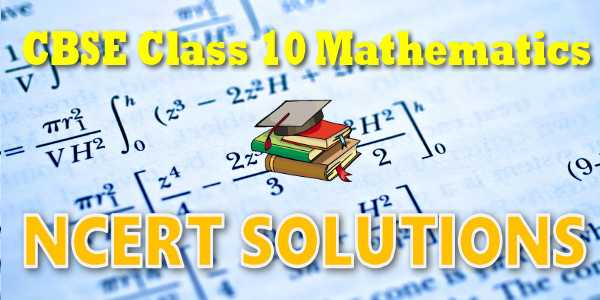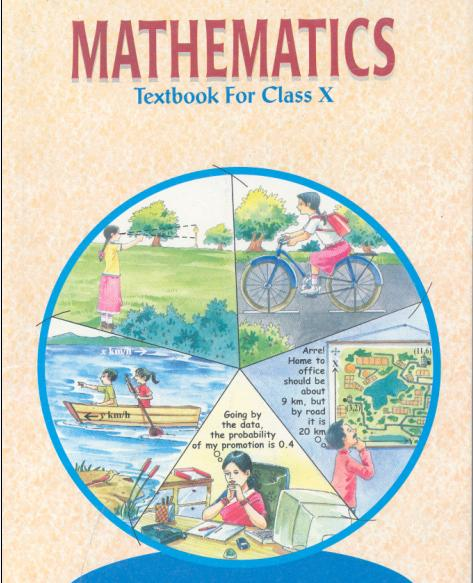1. /
2. CBSE
3. /
4. Class 10
5. /
6. Mathematics
7. /
8. NCERT Solutions for Class...

# NCERT Solutions for Class 10 Maths CBSE### myCBSEguide App

Download the app to get CBSE Sample Papers 2023-24, NCERT Solutions (Revised), Most Important Questions, Previous Year Question Bank, Mock Tests, and Detailed Notes.

NCERT books are the base books for almost all state boards in India. That’s why students across the country study NCERT textbook solutions. NCERT Solutions for Class 10 Maths includes both NCERT Maths Text Book and NCERT Maths Exemplar book. We at myCBSEguide recommend our students practice each question given in these NCERT Class 10 Maths textbooks.## NCERT Solutions for Class 10 Maths

NCERT Solutions for Class 10 Maths comprises all the questions given in the NCERT textbook. CBSE  prescribes the NCERT Maths book for class 10 in all CBSE schools. NCERT textbook questions and answers help students to get a thorough basic understanding of the concepts. It is always recommended to study NCERT books as it covers the whole syllabus. These questions with detailed explanations are now available in the myCBSEguide app for free to view and download.

NCERT Solutions for Class 10 Mathematics## Chapter-wise NCERT Solutions for Class 10 Maths

Here are chapter-wise solutions for NCERT class 10 Maths. We have included all the chapters that are given in NCERT Textbook. You can download them and save them as PDFs. We suggest you to download the myCBSEguide App to access all the NCERT textbook solutions including video solutions for each question.

### Chapter 1 – Real Numbers

Real Numbers class 10 maths NCERT solutions have four exercises. The main topics in class 10 real numbers are Fundamental Theorem of Arithmetic – statements after reviewing work done earlier and after illustrating and motivating through examples, Proofs of irrationality.

### Chapter 2 – Polynomials

NCERT Class 10th Mathematics chapter – 2 also has four exercises viz. 2.1, 2.2, 2.3 and 2.4. The topics included in Polynomials are Zeros of a polynomial. Relationship between zeros and coefficients of quadratic polynomials. Statement and simple problems on division algorithm for polynomials with real coefficients.

### Chapter 3 – Pair of Linear Equations in Two Variables

The pair of linear equations in two variables in the third chapter in class 10 NCERT book. This chapter has seven exercises. Here the topics are Pair of linear equations in two variables and the graphical method of their solution, consistency/ inconsistency. Algebraic conditions for a number of solutions. Solution of a pair of linear equations in two variables algebraically – by substitution, by elimination and by cross multiplication method. Simple situational problems. Simple problems on equations are reducible to linear equations.

### Chapter 4 – Quadratic Equations

NCERT maths solutions for the chapter quadratic equations have four subparts. It includes Standard form of a quadratic equation ax2 + bx + c = 0, (a ≠ 0). Solutions of quadratic equations (only real roots) by factorization, and by using the quadratic formula. Relationship between discriminant and nature of roots. Situational problems based on quadratic equations related to day-to-day activities to be incorporated.

### Chapter 5 – Arithmetic Progressions

Arithmetic Progressions i.e AP is one of the very interesting chapters in the class 10 Maths NCERT book. It has four exercises and the topics are Motivation for studying Arithmetic Progression Derivation of the nth term and sum of the first n terms of A.P. and their application in solving daily life problems.

### Chapter 6 – Triangles

The study of Triangles is not new for students in class 10. They have already studied some parts of this chapter in class 9 too. Here the topics are Definitions, examples, and counter examples of similar triangles. It also includes many theorems and properties.

### Chapter 7 – Coordinate Geometry

This unit has questions from lines. Concepts of coordinate geometry, graphs of linear equations. Distance formula. Section formula (internal division). Area of a triangle.

### Chapter 8 – Introduction to Trigonometry

This section has two parts Introduction and identities. It has the following topics Trigonometric ratios of an acute angle of a right-angled triangle. Proof of their existence (well defined); motivate the ratios whichever are defined at 0o and 90o. Values of the trigonometric ratios of 30, 45 and 60 degrees. Relationships between the ratios. Proof and applications of the identity sin2 A + cos2 A = 1. Only simple identities are to be given. Trigonometric ratios of complementary angles.

### Chapter 9 – Some Applications of Trigonometry

This chapter on applications of trigonometry has only one exercise. It has some simple problems on heights and distances.

### Chapter 10 – Circles

Class 10 Maths NCERT solutions on chapter circles have only a few exercises on the tangent to a circle at, the point of contact. The tangent at any point of a circle is perpendicular to the radius through the point of contact. The lengths of tangents drawn from an external point to a circle are equal.

### Chapter 11 – Constructions

The 11th chapter of class 10 maths (constructions) has only two exercises. It has questions on the division of a line segment in a given ratio, tangents to a circle from a point outside it and Construction of a triangle similar to a given triangle.

### Chapter 12 – Area Related to Circles

NCERT Class 10 maths book has an exercise on areas related to circles. This to totally based on the areas, lengths and circumference of a circle.

### Chapter 13 – Surface Areas and Volumes

Problems on the surface areas and volumes are given in exercises 13.1 to 13.5. It includes surface areas and volumes of combinations of any two of the following: cubes, cuboids, spheres, hemispheres and right circular cylinders/cones. Frustum of a cone. Problems involving converting one type of metallic solid into another and other mixed problems.

### Chapter 14 – Statistics

Although there are four exercises in class 10 maths chapter 14 (statistics) yet the scope of the problems is limited. It has the following topics Mean, median and mode of grouped data (bimodal situation to be avoided). Cumulative frequency graph.

### Chapter 15 – Probability

Probability is introduced in class 9. But students are well aware of the concepts. Here is only the Classical definition of probability and simple problems on finding the probability of an event.

## CBSE Syllabus Vs NCERT Solutions

As we know that CBSE prescribes only NCERT books for class 10 Mathematics, you will find that the CBSE syllabus and the exercises given in NCERT books are similar. Although there is a good similarity between the CBSE syllabus and NCERT books yet we advise you to go through the latest syllabus issued by CBSE and match the same with NCERT class 10 maths exercises.

### CBSE Syllabus

CBSE issues a new syllabus (curriculum document) every year. Sometimes, CBSE reduces the syllabus or adds some new topics to it. So, students must check it thoroughly and study accordingly.

### NCERT Textbooks

NCERT does not revise the textbooks every year. That’s why there may be some mismatch between the CBSE syllabus and NCERT books. Here, we suggest you to match the exercises with the CBSE syllabus before you start using it.

## NCERT Books for Class 10 Maths

CBSE Class 10 Maths has two books. CBSE has mentioned these books in its official curriculum document. So, The questions and solutions provided in these books are very important for CBSE students. These two books are:

### NCERT Maths Textbook

NCERT Class 10 Maths Textbook has 15 chapters. You can download NCERT Class 10 Maths Textbook from the official website of NCERT. It is also available chapter-wise in PDF format here.

 Chapter 1 (Open) Chapter 2 (Open) Chapter 3 (Open) Chapter 4 (Open) Chapter 5 (Open) Chapter 6 (Open) Chapter 7 (Open) Chapter 8 (Open) Chapter 9 (Open) Chapter 10 (Open) Chapter 11 (Open) Chapter 12 (Open) Chapter 13 (Open) Chapter 14 (Open) Chapter 15 (Open) Answers (Open)

### NCERT Maths Exemplar Book

In the same way, students can download the NCERT Exemplar book from NCERT’s official website for free. Here are the direct PDF file links to get the class 1o NCERT Exemplar book.

## Class 10 Mathematics PDF

NCERT Solutions Class 10 Maths PDF (Download) Free from the myCBSEguide app and myCBSEguide website. Ncert solution class 10 Maths includes textbook solutions from Mathematics Book. NCERT Solutions for CBSE Class 10 Maths has a total of 15 chapters. 10 Maths NCERT Solutions in PDF for free download on our website. Ncert Maths class 10 solutions PDF and Maths ncert class 10 PDF solutions with the latest modifications and as per the latest CBSE syllabus are only available in myCBSEguide.

## CBSE Guide App for Class 10

To download NCERT Solutions for Class 10 Maths, Computer Science, Home Science,Hindi ,English, Social Science do check myCBSEguide app or website. myCBSEguide provides sample papers with solutions, test papers for chapter-wise practice, NCERT solutions, NCERT Exemplar solutions, quick revision notes for ready reference, CBSE guess papers and CBSE important question papers. Sample Paper all are made available through the best app for CBSE students and the myCBSEguide website.Test Generator

Create question paper PDF and online tests with your own name & logo in minutes.myCBSEguide

Question Bank, Mock Tests, Exam Papers, NCERT Solutions, Sample Papers, Notes

### 12 thoughts on “NCERT Solutions for Class 10 Maths CBSE”

1. Very good

2. This is very very nice app recomended for all students

3. This is very very nice app recomended for all students

4. Thikk hai , accha hai , bus samaj me aana chaiye or kya…..

5. It is very useful to us

6. Fantastic I have learned these exercise from my text thank you

7. Very nice app. I liked it.

8. Great information! Really helpful to understand the NCER syllabus and books. Thank you

9. nice app to learn

10. 10 calass maith 1.1